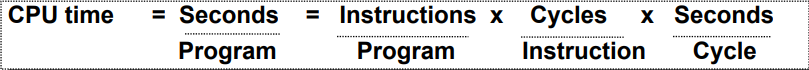Related Articles
Computer Organization | Performance of Computer
• Difficulty Level : Medium
• Last Updated : 27 Jul, 2018

Computer performance is the amount of work accomplished by a computer system. The word performance in computer performance means “How well is the computer doing the work it is supposed to do?”. It basically depends on response time, throughput and execution time of a computer system.

Response time is the time from start to completion of a task. This also includes:

• Waiting for I/O and other processes
• Accessing disk and memory
• Time spent executing on the CPU or execution time.

Throughput is the total amount of work done in a given time.

CPU execution time is the total time a CPU spends computing on a given task. It also excludes time for I/O or running other programs. This is also referred to as simply CPU time.

Performance is determined by execution time as performance is inversely proportional to execution time.

`Performance = (1 / Execution time) `

And,

```(Performance of A /  Performance of B)
= (Execution Time of B / Execution Time of A) ```

If given that Processor A is faster than processor B, that means execution time of A is less than that of execution time of B. Therefore, performance of A is greater than that of performance of B.

Example –
Machine A runs a program in 100 seconds, Machine B runs the same program in 125 seconds

```(Performance of A /  Performance of B)
= (Execution Time of B / Execution Time of A)
= 125 / 100 = 1.25 ```

That means machine A is 1.25 times faster than Machine B.

And, the time to execute a given program can be computed as:

`Execution time  = CPU clock cycles x clock cycle time `

Since clock cycle time and clock rate are reciprocals, so,

`Execution time  = CPU clock cycles / clock rate `

The number of CPU clock cycles can be determined by,

```CPU clock cycles
= (No. of instructions / Program ) x (Clock cycles / Instruction)
= Instruction Count x CPI ```

Which gives,

```Execution time
= Instruction Count x CPI x clock cycle time
= Instruction Count x CPI / clock rate  ```

The units for CPU Execution time are:How to Improve Performance?
To improve performance you can either:

• Decrease the CPI (clock cycles per instruction) by using new Hardware.
• Decrease the clock time or Increase clock rate by reducing propagation delays or by use pipelining.
• Decrease the number of required cycles or improve ISA or Compiler.
My Personal Notes arrow_drop_up